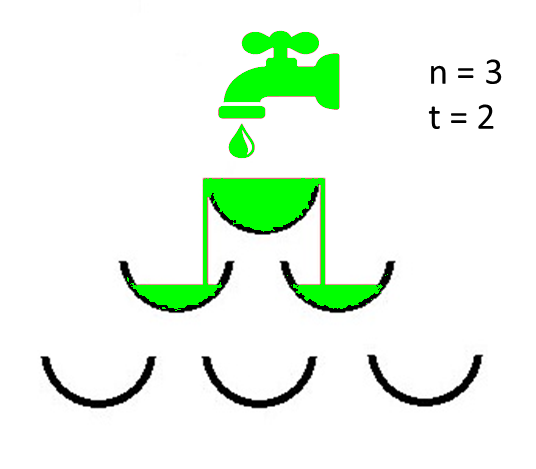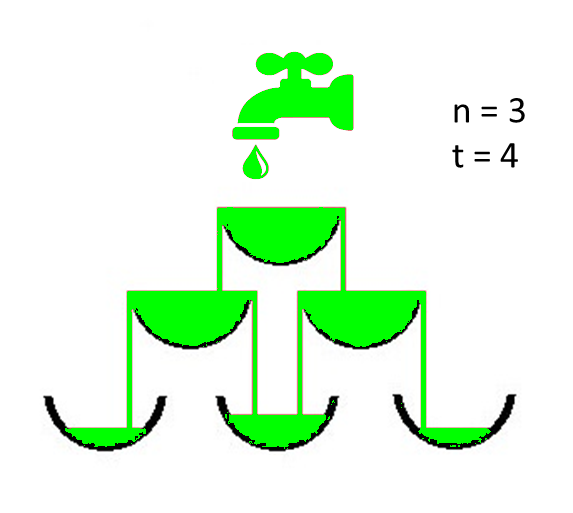Related Articles

# Count of vessels completely filled after a given time

• Difficulty Level : Expert
• Last Updated : 14 Jun, 2021

Given two integers N and T denoting the number of levels and the number of seconds respectively, the task is to find the number of completely filled vessels after T seconds under given conditions:

• A structure of vessels of N levels is such that the number of the vessels at each level is equal to the level number i.e 1, 2, 3, … up to N.
• Each vessel can store a maximum of 1 unit of water and in every second 1 unit water is poured out from a tap at a constant rate.
• If the vessel becomes full, then water starts flowing out of it, and pours over the edges of the vessel, and is equally distributed over the two connected vessels immediately below it.

Assumptions:

1. All the objects are arranged symmetrically along the horizontal axis.
2. All levels are equally spaced.
3. Water flows symmetrically over both the edges of the vessel.

Examples:

Input: N = 3, T = 2
Output: 1
Explanation:
View of Structure with N = 3 and at a time T = 2 after the tap has been openedInput: N = 3, T = 4
Output: 3
Explanation:
View of Structure with N = 3 and at a time T = 4 after the tap has been openedNaive Approach: The simplest approach to solve the problem is to check if it is possible to completely fill x vessels in T seconds or not. If found to be true, check for x+1 vessels and repeat so on to obtain the maximum value of x.
Time Complexity: O(N3)
Auxiliary Space: O(1)

Efficient Approach: The above approach can be optimized using Dynamic Programming. Follow the steps below to solve the problem:

1. Store the vessel structure in a Matrix, say M, where M[i][j] denotes the jth vessel in the ith level.
2. For any vessel M[i][j], the connected vessels at an immediately lower level are M[i + 1][j] and M[i + 1][j + 1].
3. Initially, put all water in the first vessel i, e. M = t.
4. Recalculate the state of the matrix at every increment of unit time, starting from the topmost vessel i, e. M = t.
5. If the amount of water exceeds the volume of the vessel, the amount flowing down from a vessel is split into 2 equal
6. parts filling the two connected vessels at immediately lower level.

## C++

 `// C++ program to implement ``// the above approach ``#include  ``using` `namespace` `std; ``  ` `int` `n, t;``  ` `// Function to find the number of``// completely filled vessels``int` `FindNoOfFullVessels(``int` `n, ``int` `t)``{``      ` `    ``// Store the vessels``    ``double` `Matrix[n][n];``  ` `    ``// Assuming all water is present``    ``// in the vessel at the first level``    ``Matrix = t * 1.0;``  ` `    ``// Store the number of vessel``    ``// that are completely full``    ``int` `ans = 0;``  ` `    ``// Traverse all the levels``    ``for``(``int` `i = 0; i < n; i++)``    ``{``          ` `        ``// Number of vessel at each``        ``// level is j``        ``for``(``int` `j = 0; j <= i; j++)``        ``{``              ` `            ``// Calculate the exceeded``            ``// amount of water``            ``double` `exceededwater = Matrix[i][j] - 1.0;``  ` `            ``// If current vessel has``            ``// less than 1 unit of``            ``// water then continue``            ``if` `(exceededwater < 0)``                ``continue``;``  ` `            ``// One more vessel is full``            ``ans++;``  ` `            ``// If left bottom vessel present``            ``if` `(i + 1 < n)``                ``Matrix[i + 1][j] += exceededwater / 2;``  ` `            ``// If right bottom vessel present``            ``if` `(i + 1 < n && j + 1 < n)``                ``Matrix[i + 1][j + 1] += exceededwater / 2;``        ``}``    ``}``    ``return` `ans;``}``  ` `// Driver Code``int` `main()``{``      ` `    ``// Number of levels``    ``int` `N = 3;``  ` `    ``// Number of seconds``    ``int` `T = 4;``  ` `    ``// Function call``    ``cout << FindNoOfFullVessels(N, T) << endl;``      ` `    ``return` `0;``}``  ` `// This code is contributed by sanjoy_62`

## Java

 `// Java Program to implement``// the above approach``  ` `import` `java.io.*;``import` `java.util.*;``  ` `class` `GFG {``  ` `    ``static` `int` `n, t;``  ` `    ``// Function to find the number of``    ``// completely filled vessels``    ``public` `static` `int``    ``FindNoOfFullVessels(``int` `n, ``int` `t)``    ``{``        ``// Store the vessels``        ``double` `Matrix[][]``            ``= ``new` `double``[n][n];``  ` `        ``// Assuming all water is present``        ``// in the vessel at the first level``        ``Matrix[``0``][``0``] = t * ``1.0``;``  ` `        ``// Store the number of vessel``        ``// that are completely full``        ``int` `ans = ``0``;``  ` `        ``// Traverse all the levels``        ``for` `(``int` `i = ``0``; i < n; i++) {``  ` `            ``// Number of vessel at each``            ``// level is j``            ``for` `(``int` `j = ``0``; j <= i; j++) {``  ` `                ``// Calculate the exceeded``                ``// amount of water``                ``double` `exceededwater``                    ``= Matrix[i][j] - ``1.0``;``  ` `                ``// If current vessel has``                ``// less than 1 unit of``                ``// water then continue``                ``if` `(exceededwater < ``0``)``                    ``continue``;``  ` `                ``// One more vessel is full``                ``ans++;``  ` `                ``// If left bottom vessel present``                ``if` `(i + ``1` `< n)``                    ``Matrix[i + ``1``][j]``                        ``+= exceededwater / ``2``;``  ` `                ``// If right bottom vessel present``                ``if` `(i + ``1` `< n && j + ``1` `< n)``                    ``Matrix[i + ``1``][j + ``1``]``                        ``+= exceededwater / ``2``;``            ``}``        ``}``  ` `        ``return` `ans;``    ``}``  ` `    ``// Driver Code``    ``public` `static` `void` `main(String[] args)``    ``{``        ``// Number of levels``        ``int` `N = ``3``;``  ` `        ``// Number of seconds``        ``int` `T = ``4``;``  ` `        ``// Function call``        ``System.out.println(``            ``FindNoOfFullVessels(N, T));``    ``}``}`

## Python3

 `# Python3 program to implement    ``# the above approach    ``     ` `# Function to find the number of    ``# completely filled vessels    ``def` `FindNoOfFullVessels(n, t) :    ``        ` `    ``# Store the vessels    ``    ``Matrix ``=` `[[``0` `for` `i ``in` `range``(n)] ``for` `j ``in` `range``(n)]   ``     ` `    ``# Assuming all water is present    ``    ``# in the vessel at the first level    ``    ``Matrix[``0``][``0``] ``=` `t ``*` `1.0`    `     ` `    ``# Store the number of vessel    ``    ``# that are completely full    ``    ``ans ``=` `0`    `     ` `    ``# Traverse all the levels    ``    ``for` `i ``in` `range``(n) :   ``            ` `        ``# Number of vessel at each    ``        ``# level is j    ``        ``for` `j ``in` `range``(i ``+` `1``) :   ``                ` `            ``# Calculate the exceeded    ``            ``# amount of water    ``            ``exceededwater ``=` `Matrix[i][j] ``-` `1.0`    `     ` `            ``# If current vessel has    ``            ``# less than 1 unit of    ``            ``# water then continue    ``            ``if` `(exceededwater < ``0``) :   ``                ``continue`   `     ` `            ``# One more vessel is full    ``            ``ans ``+``=` `1`    `     ` `            ``# If left bottom vessel present    ``            ``if` `(i ``+` `1` `< n) :    ``                ``Matrix[i ``+` `1``][j] ``+``=` `exceededwater ``/` `2`   `     ` `            ``# If right bottom vessel present    ``            ``if` `(i ``+` `1` `< n ``and` `j ``+` `1` `< n) :   ``                ``Matrix[i ``+` `1``][j ``+` `1``] ``+``=` `exceededwater ``/` `2`    `    ``return` `ans   ``     ` `        ` `# Number of levels    ``N ``=` `3`   `     ` `# Number of seconds    ``T ``=` `4`   `     ` `# Function call    ``print``(FindNoOfFullVessels(N, T))` `# This code is contributed by divyesh072019`

## C#

 `// C# program to implement ``// the above approach ``using` `System; ``  ` `class` `GFG{``  ` `//static int n, t;``  ` `// Function to find the number of``// completely filled vessels``public` `static` `int` `FindNoOfFullVessels(``int` `n, ``                                      ``int` `t)``{``      ` `    ``// Store the vessels``    ``double``[,] Matrix = ``new` `double``[n, n];``  ` `    ``// Assuming all water is present``    ``// in the vessel at the first level``    ``Matrix[0, 0] = t * 1.0;``  ` `    ``// Store the number of vessel``    ``// that are completely full``    ``int` `ans = 0;``  ` `    ``// Traverse all the levels``    ``for``(``int` `i = 0; i < n; i++)``    ``{``  ` `        ``// Number of vessel at each``        ``// level is j``        ``for``(``int` `j = 0; j <= i; j++) ``        ``{``  ` `            ``// Calculate the exceeded``            ``// amount of water``            ``double` `exceededwater = Matrix[i, j] - 1.0;``  ` `            ``// If current vessel has``            ``// less than 1 unit of``            ``// water then continue``            ``if` `(exceededwater < 0)``                ``continue``;``  ` `            ``// One more vessel is full``            ``ans++;``  ` `            ``// If left bottom vessel present``            ``if` `(i + 1 < n)``                ``Matrix[i + 1, j] += exceededwater / 2;``  ` `            ``// If right bottom vessel present``            ``if` `(i + 1 < n && j + 1 < n)``                ``Matrix[i + 1, j + 1] += exceededwater / 2;``        ``}``    ``}``    ``return` `ans;``}``  ` `// Driver Code``public` `static` `void` `Main()``{``      ` `    ``// Number of levels``    ``int` `N = 3;``  ` `    ``// Number of seconds``    ``int` `T = 4;``  ` `    ``// Function call``    ``Console.WriteLine(FindNoOfFullVessels(N, T));``}``}``  ` `// This code is contributed by sanjoy_62`

## Javascript

 ``

Output:

`3`

Time complexity: O(N2)
Space Complexity: O(N2)My Personal Notes arrow_drop_up